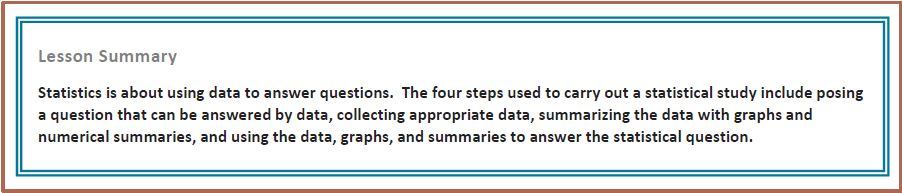6th Module 6--Statistics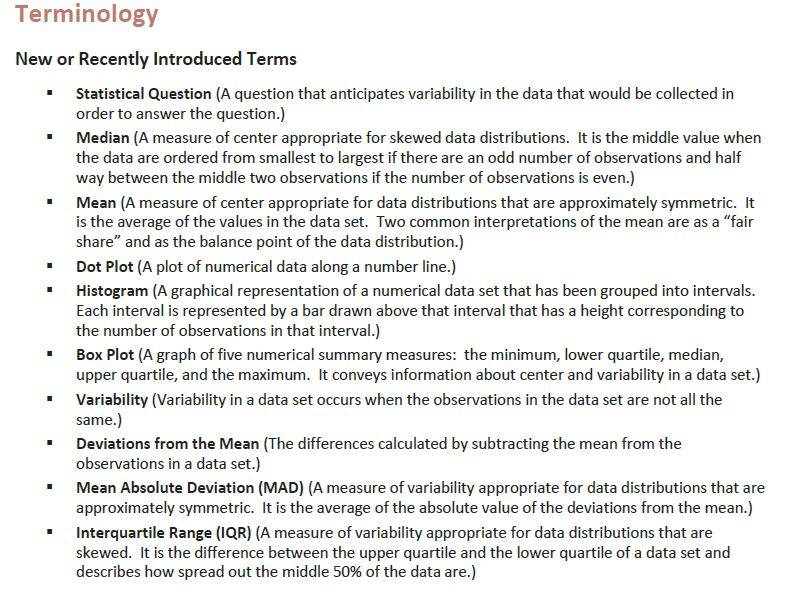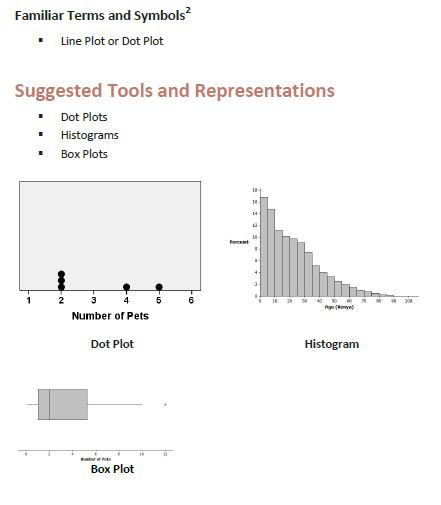Topic A: Understanding Distributions

Statistical and non statistical questions:

Practice:
R.11  Create histograms R.6    Create frequency tables

Topic B: Summarizing a Distribution that is Approximately Symmetric Using the Mean and Mean Absolute Deviation

Exploring mean and median module: Ben Eater and Sal work through the mean and median module: http://www.khanacademy.org/math/statistics/e/exploring_mean_and_median

Practice:

S.4  Identify representative, random, and biased samples

Lesson 6-'Fair Share'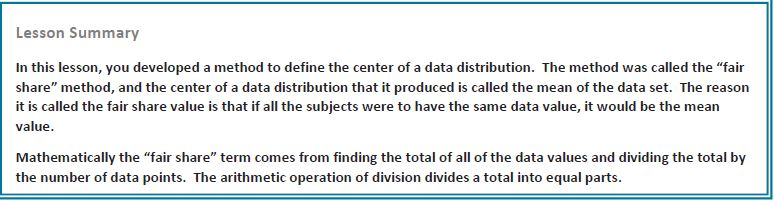Lesson 7: The Mean as a Balance Point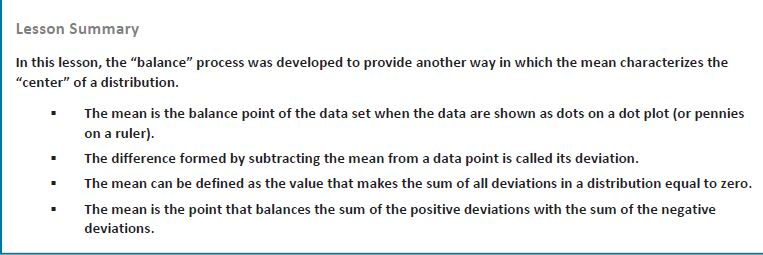Lesson 8: Variability in a Data Distribution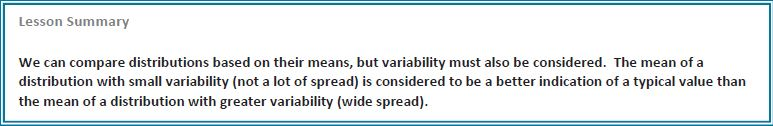Lesson 9: The Mean Absolute Deviation (MAD)Lesson 10: Describing Distributions Using the Mean and MADLesson 11: Describing Distributions Using the Mean and MAD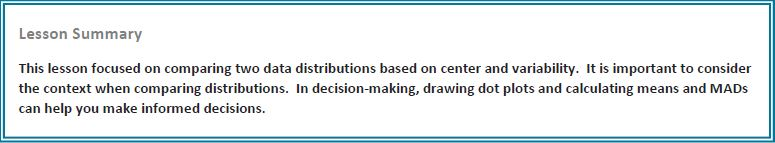Topic C: Summarizing a Distribution that is Skewed Using the Median and the Interquartile Range

Practice:

R.17  Interpret box-and-whisker plots
S.1    Calculate mean, median, mode, and range
S.2     Interpret charts to find mean, median, mode, and range

Lesson 12: Describing the Center of a Distribution Using the Median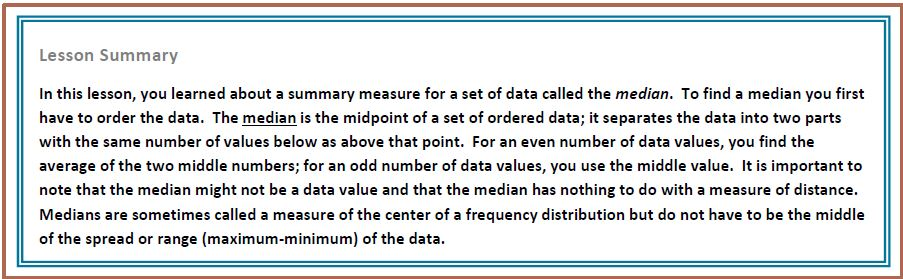Lesson 13:  Describing Variability Using the Interquartile Range (IQR)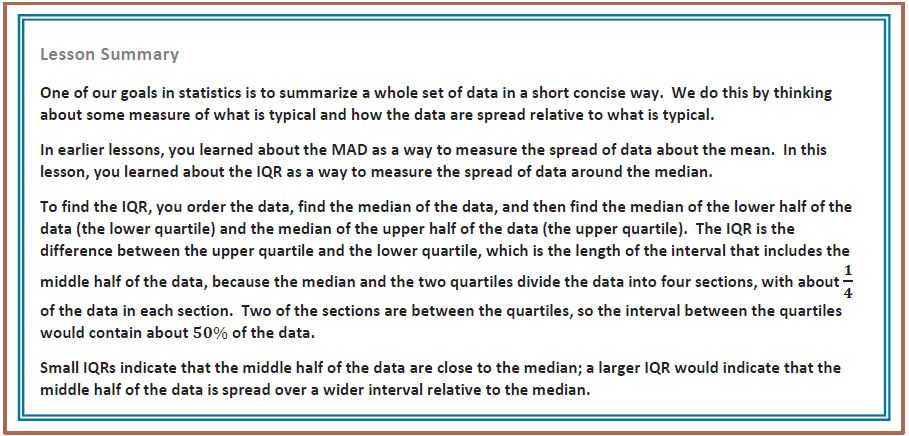Lesson 14: Summarizing a Distribution Using a Box Plot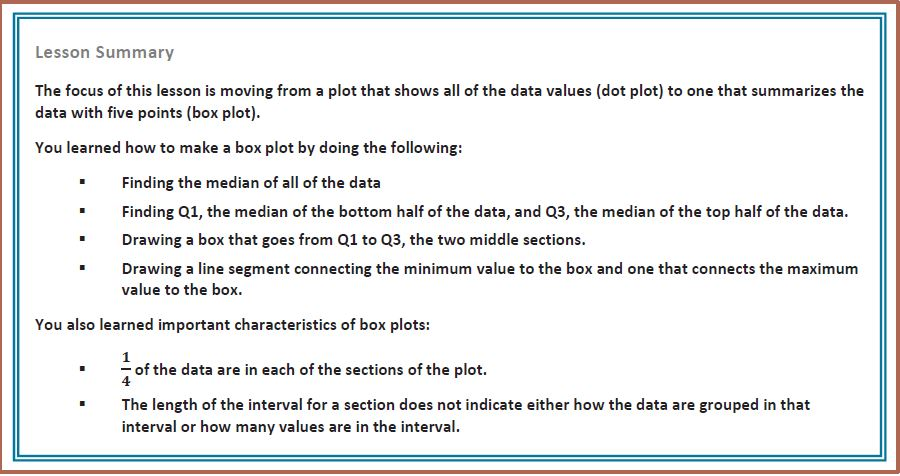Lesson 15: More Practice with Box PlotsLesson 16: Understanding Box Plots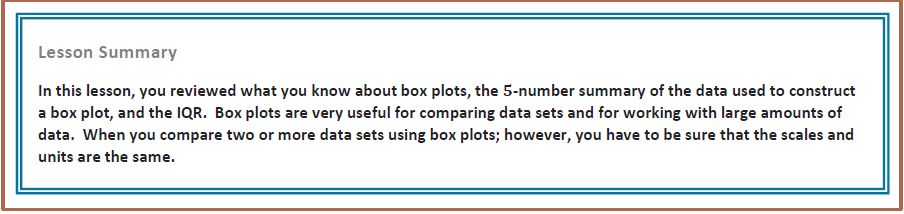Topic D: Summarizing and Describing Distributions

Lesson 17: Developing a Statistical Project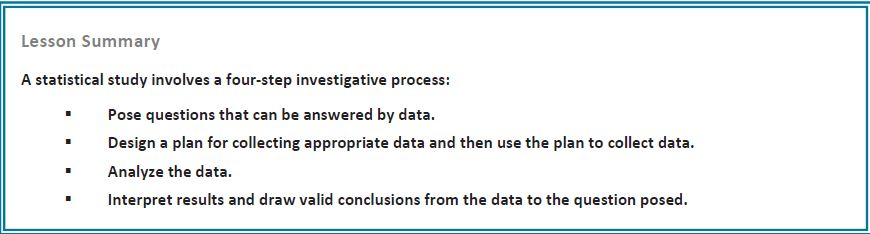Lesson 18: Connecting Graphical Representations and Numerical Summaries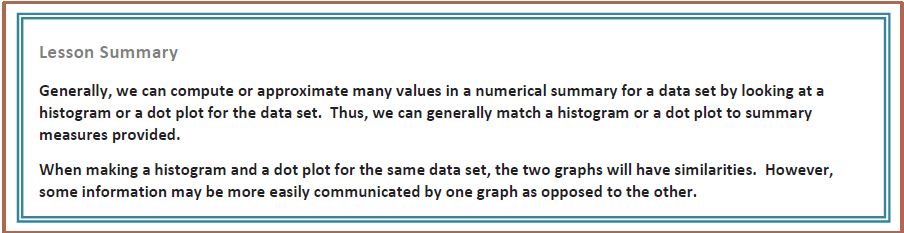Lesson 19: Comparing Data DistributionsLesson 20: Describing Center, Variablitiy, and a Shape of a Data Distribution from a Graphical RepresentationLesson 21: Summarizing a Data Distribution by Describing Center, Variability, and ShapeLesson 22: Presenting a Summary of a Statistical Project